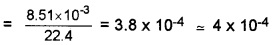# Plus One Physics Chapter Wise Questions and Answers Chapter 13 Kinetic Theory

Students can Download Chapter 13 Kinetic Theory Questions and Answers, Plus One Physics Chapter Wise Questions and Answers helps you to revise the complete Kerala State Syllabus and score more marks in your examinations.

## Kerala Plus One Physics Chapter Wise Questions and Answers Chapter 13 Kinetic Theory

### Plus One Physics Kinetic Theory One Mark Questions and Answers

Question 1.
The value of $$\frac{P V}{T}$$ for one mole of an ideal gas is nearly equal to
(a) 2 Jmol-1K-1
(b) 8.3 Jmol-1K-1
(c) 4.2 Jmol-1K-1
(d) 2 cal mol-1K-1
(d) 2 cal mol-1K-1
The value of $$\frac{P V}{T}$$ for one mole of an ideal gas = gas constant = 2 cal mol-1K-1.

Question 2.
Mean free path of a gas molecule is
(a) inversely proportional to number of molecules per unit volume
(b) inversely proportional to diameter of the molecule
(c) directly proportional to the square root of the absolute temperature
(d) directly proportional to the molecular mas
(a) inversely proportional to number of molecules per unit volume.Question 3.
If for a gas $$\frac{R}{C_{v}}$$ = 0.67, this gas is made up of molecules which are
(a) monoatomic
(b) diatomic
(c) Polyatomic
(d) mixture of diatomic and polyatomic molecules
(a) monoatomic
For a gas, we know $$\frac{R}{C_{v}}$$ = γ – 1
or, 0.67 = γ – 1, or γ = 1.67
Hence the gas is monoatomic.

Question 4.
According to kinetic theory of gases, molecules of a gas behave like
(a) inelastic spheres
(b) perfectly elastic rigid spheres
(c) perfectly elastic non-rigid spheres
(d) inelastic non-rigid spheres
(b) According to kinetic theory of gases, gas molecules behave as a perfectly elastic rigid spheres.

Question 5.
Which one of the following is not an assumption of kinetic theory of gases?
(a) The volume occupied by the molecules of the gas is negligible.
(b) The force of attraction between the molecules is negligible.
(c) the collision between the molecules are elastic.
(d) All molecules have same speed.
(d) Molecules of an ideal gas moves randomly with different speeds.

Question 6.
What is the shape of graph between volume and temperature, if pressure is kept constant?
PV = nRT. Hence graph will be straight line.

Question 7.
What is the shape of graph between pressure p and I/V for a perfect gas at constant temperature?
Straight lineQuestion 8.
Identify the minimum possible temperature at which all molecular motion ceases.
Absolute temperature (OK or – 273.15°C).

Question 9.
What is the formula for average translational kinetic energy of a gas molecule?
3/2 KBT

### Plus One Physics Kinetic Theory Two Mark Questions and Answers

Question 1.
Mention the conditions under which the real gases obey ideal gas equation.
Low pressure and high temperature.

Question 2.
Why the temperature rises when gas is suddenly compressed?
The work done on gas during compression increases the kinetic energy of molecules and hence temperature of gas rises.Question 3.
Why evaporation causes cooking?
During evaporation, fast moving molecules escape from liquid. Hence average kinetic energy of molecules left behind is decreaesd. This will reduce temperature & causes cooling.

Question 4.
When automobile travels long distance air pressure in tyres increases slightly. Why?
As automobile moves, work is being done against force of friction. This work is converted in to heat and it increases the temperature. As P a T, increase in temperature will increase pressure.

Question 5.
PV = µ RT is the ideal gas equation. Real gas obeys ideal behaviour at high temperature and at low pressure.

1. Give an example for ideal gas
2. Why real gases obey ideal gas equation at high temperature and at low pressure.

1. Hydrogen
2. The interaction between molecules can be neglected at high T and at low temperature.Question 6.
A vessel of volume V contains a gas of µ moles at a temperature T.

1. What is the ideal gas equation if gas is considered to be ideal one?
2. The variation of pressure P with number of moles per unit volume in a vessel is shown in the graph. Analyse the graph and choose the correct one and justify your answer.(i) The temperature inside the vessel decreases.
(ii) The temperature inside the vessel increases.
1. PV = µRT

2. P = $$\frac{1}{3}$$nmc-2
In this case, when n increases, (mc-2) decreases to maintain P as constant. Temperature is directly proportional to mc-2. Hence we can say that temperature inside the vessel decreases.

### Plus One Physics Kinetic Theory Three Mark Questions and Answers

Question 1.
1 mole of ideal gas is taken in vessel.

1. State the following statements as true or false.
• In gas equation R is constant.
• All real gas obeys gas equation at all temperature and pressures.
2. Draw the variation of R with pressure for the above ideal gas.
3. Draw the variation of R with volume for this ideal gas.

1. Following statements as true or false:

• True
• False

2.3.Question 2.

1. Air pressure in a car tyre increase during driving. Why?
2. Air is filled in a vessel at 60°C. To what temperature should it be heated in order that 1/3rd of air may escape out of the vessel? (Expansion of air may be neglected).

1. During driving the temperature of air inside the tyre increases due to motion.

2. T1 = 60 + 273 = 333K
V1 = V; T2 = ? V2 = V+ V/3
V2 = $$\frac{4}{3}$$VQuestion 3.
Find the degrees of freedom of the following.

1. A body is confined to move in a straight line
2. A body moves in a plane
3. A body moves in a space

1. 1
2. 2
3. 3

### Plus One Physics Kinetic Theory Four Mark Questions and Answers

Question 1.
An enclosed vessel contains many number of molecules moving in random direction.

1. Explain the term pressure in terms molecular concept.
2. Derive an expression for the pressure exerted by the gas molecules by assuming postulates of kinetic theory of gases.

1. Pressure P = $$\frac{2}{3} n \overline{K E}$$
Where ‘n’ is the number of gas molecules per unit volume. $$\overline{\mathrm{KE}}$$ is the average kinetic energy of a gas molecules moving in random direction.

2.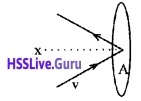Consider molecules of gas in a container. The molecules are moving in random directions with a velocity V. This is the velocity of a molecule in any direction.

The velocity V can be resolved along x, y and z directions as Vx, Vy, and Vz respectively. If we assume a molecule hits the area A of container with velocity Vx and rebounds back with -Vx.

The change in momentum imparted to the area A by the molecule = 2mVx. The molecules covers a distance Vxt along the x-direction in a time t. All the molecules within the volume AVxt will collide with the area in a time t.

If ‘n’ is the number of molecules per unit volume, the total number of molecules hitting the area A, N = AVxt n.
But on an average, only half of those molecules will be hitting the area, and the remaining molecules will be moving away from the area. Hence the momentum imported to the area in a time t.Q = 2mvx × $$\frac{1}{2}$$ AVxt n.
= nmVx2 At
The rate of change of momentum,
$$\frac{Q}{t}$$ = nmVx2 A
But rate of change of momentum is called force, ie. force F = nmVx2A
∴ pressure P =nmVx 2 (P = $$\frac{F}{A}$$)
Different molecules move with different velocities. Therefore, the average value V2x is to be taken. If $$\overline{\mathbf{V}}_{\mathbf{x}}^{2}$$ isthe average value then the pressure.
p = nm$$\overline{\mathbf{V}}_{\mathbf{x}}^{2}$$ ………(1)
$$\overline{\mathbf{V}}_{\mathbf{x}}^{2}$$ is known as the mean square velocity.
Since the gas is isotropic (having the same properties in all directions), we can writeHence the eq (1) can be written as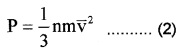But nm = ρ, the density of gas
∴ P = $$\frac{F}{A}$$ ρ$$\overline{\mathbf{V}}^{2}$$.Question 2.
1. Fill in the blanks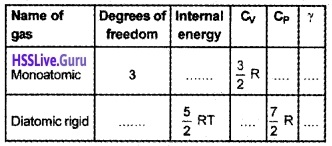2. What happens to the value of ratio of specific heat capacity, if we consider all rotational degrees of freedom of a 1-mole diatomic molecule?
1.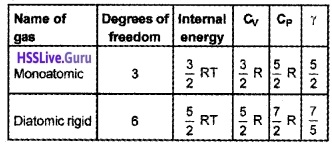2. Total degrees of freedom = 3 (trans) + 3 (Rot) = 6
∴ CV = 3R, CP = 4R
Ratio of specific heat γ = $$\frac{4}{3}$$
Ratio of specific heat capacity decreases.

### Plus One Physics Kinetic Theory NCERT Questions and Answers

Question 1.
Molar volume is the volume occupied by 1 mol of any (ideal) gas at standard temperature and pressure (STP: 1 atmospheric pressure, 0°C). Show that it is 22.4 litres.
PV= µRT or V = $$\frac{\mu \mathrm{RT}}{\mathrm{P}}$$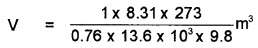= 22.4 × 10-3 m3 = 22.4 litre.

Question 2.
Estimate the total number of air molecules (inclusive of oxygen, nitrogen, water vapour and other constituents) in a room of capacity 25.0m3 at a temperature of 27°C and 1-atmosphere pressure.
V = 25.0m3, T = (27 + 273), K = 300 K, k = 1.38 × 10-23JK-1
PV= nRT = n(Nk)T = (nN)kT = NtkT
Here Nt represents the total number of air molecules in the given gas.Question 3.
From a certain apparatus, the diffusion rate of hydrogen has an average value of 28.7 cm3s-1. The diffusion of another gas under the same conditions is measured to have an average rate of 7.2 cm3s-1. Identify the gas.
According to Graham’s law of diffusion of gases, the rate of diffusion of a gas is inversely proportional to the square root of its molecular mass. If R1 and R2 be the rates of diffusion of two gases having molecular masses M1 and M2 respectively, then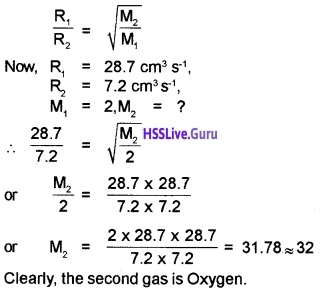Question 4.
Estimate the fraction of molecular volume to the actual volume occupied by oxygen gas at STP. Take the diameter of an oxygen molecule to be 3Å.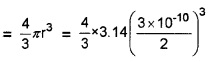= $$\frac{4}{3}$$ × 3.142(1.5)3 × 10-30 × 6.02 × 1023 m3